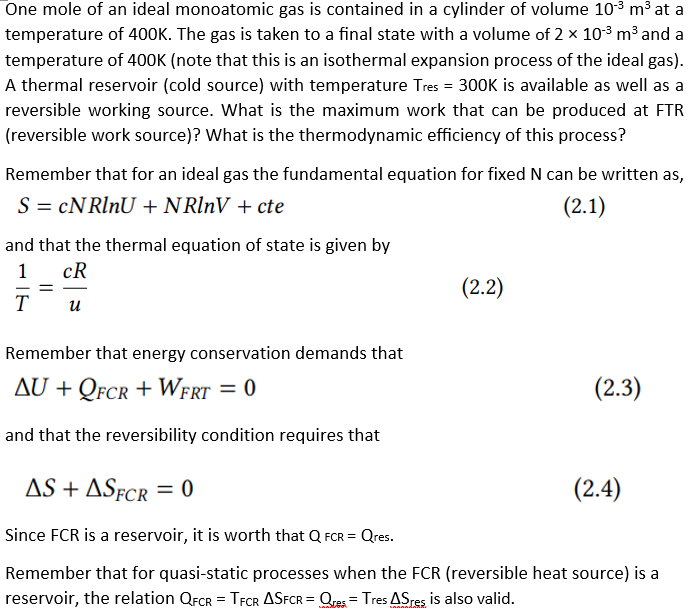Home / Expert Answers / Chemical Engineering / one-mole-of-an-ideal-monoatomic-gas-is-contained-in-a-cylinder-of-volume-10-pa865

# (Solved): One mole of an ideal monoatomic gas is contained in a cylinder of volume 10 ...​​​​​​​

One mole of an ideal monoatomic gas is contained in a cylinder of volume at a temperature of . The gas is taken to a final state with a volume of and a temperature of (note that this is an isothermal expansion process of the ideal gas). A thermal reservoir (cold source) with temperature Tres is available as well as a reversible working source. What is the maximum work that can be produced at FTR (reversible work source)? What is the thermodynamic efficiency of this process? Remember that for an ideal gas the fundamental equation for fixed can be written as, and that the thermal equation of state is given by Remember that energy conservation demands that and that the reversibility condition requires that Since FCR is a reservoir, it is worth that . Remember that for quasi-static processes when the FCR (reversible heat source) is a reservoir, the relation is also valid.

We have an Answer from Expert